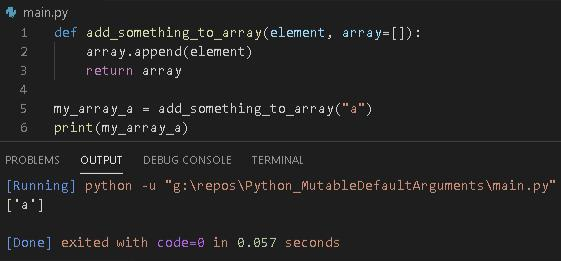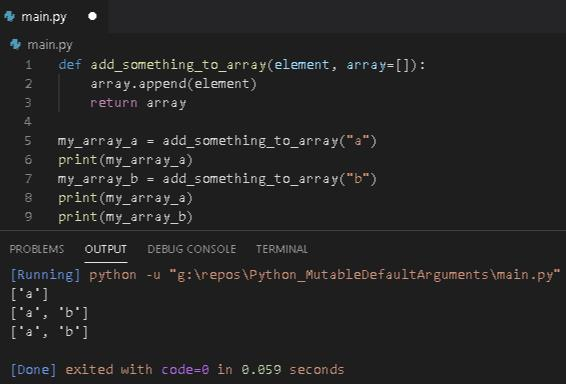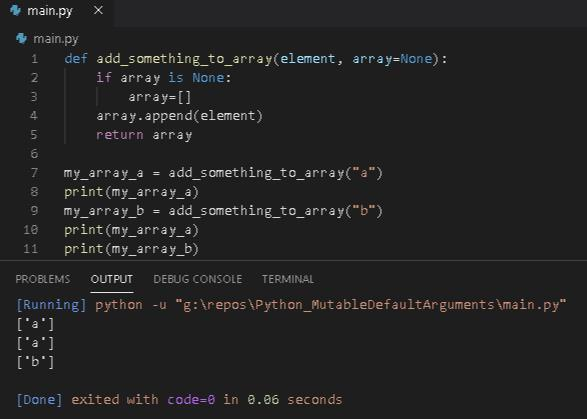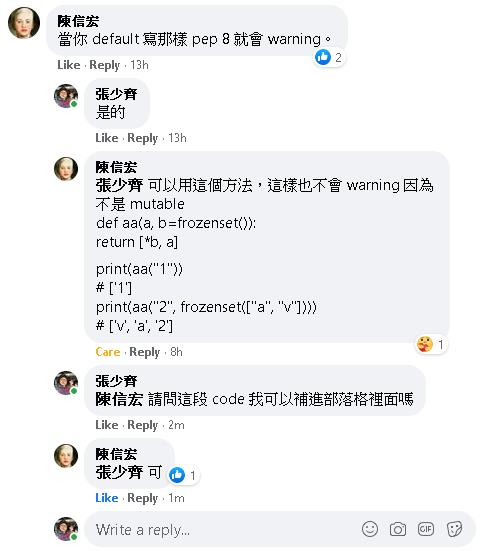### Python - Mutable Default Arguments 筆記

def add_something_to_array(element, array=[]):
array.append(element)
return array

print(my_array_a)## 但是

def add_something_to_array(element, array=[]):
array.append(element)
return array

print(my_array_a)
print(my_array_a)
print(my_array_b)Python 在定義 function 的預設參數時，只會被定義一次，而不是在每次呼叫的時候重新定義一次，所以上面這一小段 code: array = [] 才會一直被重複使用。

## 那該怎麼做呢?

def add_something_to_array(element, array=None):
if array is None:
array=[]
array.append(element)
return array

print(my_array_a)
print(my_array_a)
print(my_array_b)## 感謝網友陳信宏補充，使用 frozenset() 也能夠避免此 code lint 警告

def aa(a, b=frozenset()):
return [*b, a]
print(aa("1"))
# ['1']
print(aa("2", frozenset(["a", "v"])))
# ['v', 'a', '2']